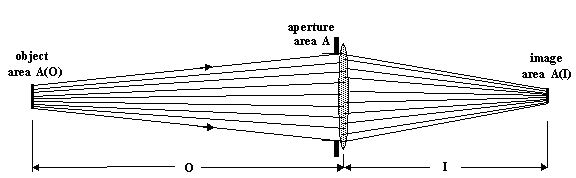# The f-number

Consider a thin, symmetric, circular, bi-convex lens of focal length F, and a circular aperture having area A (diameter D). A small object of area A(O) is a distance O in front of the lens. Its image, which is behind the lens, has area A(I).B*A(O)*A/O^2

E=(BA/O^2)*A(O)/A(I)

E=BA/(MO)^2

M=F/(O-F) ==> MO=F(M+1)

E=(pi)B/(4f^2)

f=(M+1)(F/D)